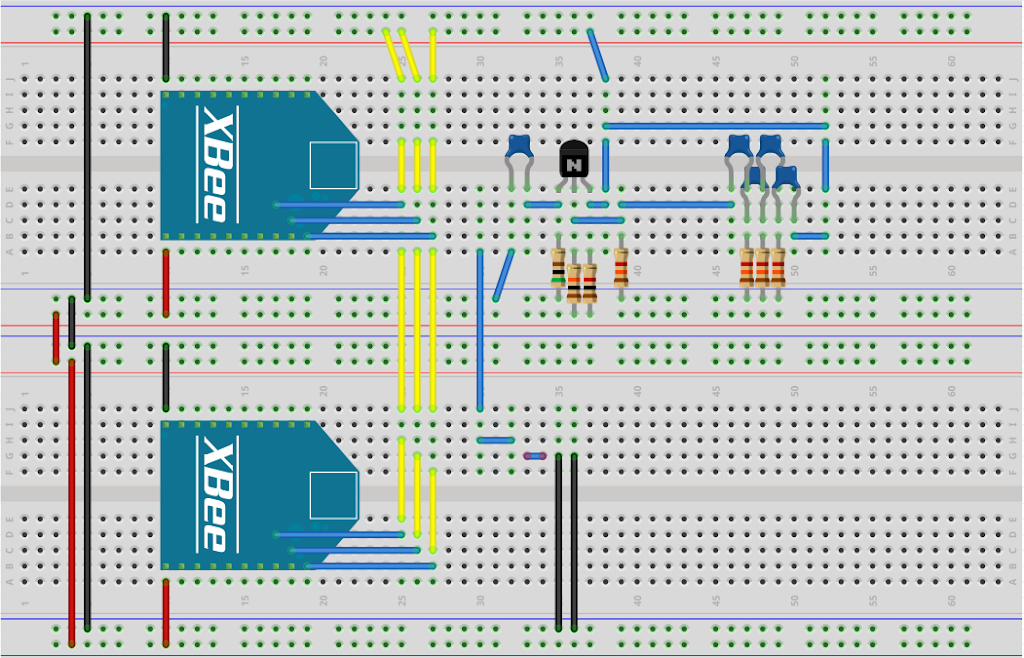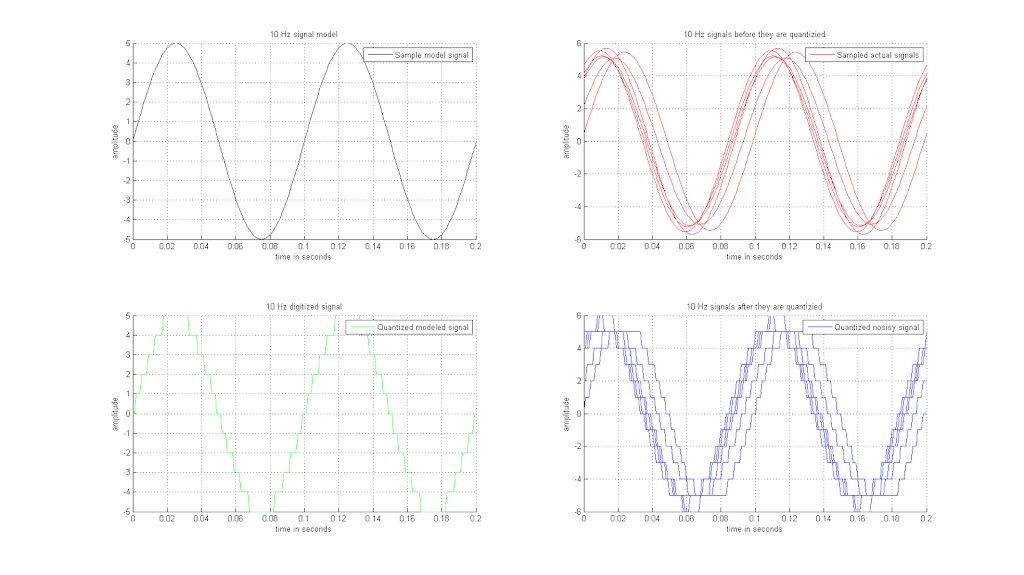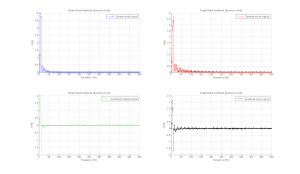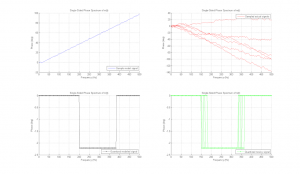Posted on

# Do not go gentle into that good night

Dylan Thomas1914 – 1953

Do not go gentle into that good night,
Old age should burn and rave at close of day;
Rage, rage against the dying of the light.

Though wise men at their end know dark is right,
Because their words had forked no lightning they
Do not go gentle into that good night.

Good men, the last wave by, crying how bright
Their frail deeds might have danced in a green bay,
Rage, rage against the dying of the light.

Wild men who caught and sang the sun in flight,
And learn, too late, they grieved it on its way,
Do not go gentle into that good night.

Grave men, near death, who see with blinding sight
Blind eyes could blaze like meteors and be gay,
Rage, rage against the dying of the light.

And you, my father, there on the sad height,
Curse, bless, me now with your fierce tears, I pray.
Do not go gentle into that good night.
Rage, rage against the dying of the light.

Posted on

## Fun with XBees and Jitter 2 of 4: SimulationIntroduction:
Now that we think we know what will happen when we sample our output lets build it!  I need a way to generate the signal for the Xbee to measure. While I have a function generator I realized these may not be common or they may be to expensive so I included the methodology to design a function with a circuit below. But first the high level breadboard design.

I made this diagram using a really cool program from fritzing. Below are two Series 2 Xbees (from Digi) with the first 3 ports of each Xbee connected to the analog signal output [D0:2] for 6 samples total. The red wires are power (5V) and the black wires are ground. The other colors represent signals. The resistors are the correct colors, thanks to fritzing! This design is pretty basic but it is intended to be this way. Ideally it will give enough simplicity to do a robust analysis and also show some utility.
The Xbee’s quantize these signals then transmit them across the mesh. Can these signals be synchronized and reconstructed synthetically? Does a mesh architecture lend efficacy to the reconstruction of a signal? Was Nyquist right? And in doing so will be gain more bandwidth from these XBees? What is bandwidth anyways…and why do we need it so badly? Maybe… 🙂 Well see in future blog posts.Figure 1: The transmitter bread board. This circuit will  first convert an analog signal  generated by the BJT Phase Shift Oscillator to a digital signal then transmit the signal to the receiver board. In this case it is an FEZ Cerbuino from GHI with another series 2 Xbee and serial port attached.

XBee Series 2 Configuration:
I configured the XBee series 2 modules to be in API mode and act as routers. I decided to choose routers rather than endpoints so they could communicate to each other as well as the coordinator. To set up the XBees I used Digi’s tool: XCTU, it is free and works really well for with the XBees. Both Xbees are configured exactly the same by loading the same profile into both of them.

BJT Phase shift Oscillator:
Here is a inexpensive and easy way to build a function generator the function this circuit generates is:
[m(t)=(5+epsilon (t))sinleft(2pi 887+phi (t))]

Schematic:
I used EasyEDA to design this circuit. It is a really nice tool for simple and quick circuits and PCB layout as well as a potential PCB supply source. Well worth the price. Here is my design:

PCB:

Analysis:

The first step is to break this cirucit down into two sub-circuits. The frequency attenuation circuit and the amplifier (BJT) circuit.Next we need to make a few assumptions. For the BJT I’m going to use the Fairchild datasheet. Here are my assumptions and givens:
• Current gain: >= 100 @ 150mA. Because the voltage is lower than the spec sheet recommends the gain is probably closer to 35.
• Power supply voltage: 5V
• Disapation power of 50mW
• Desire a 1 kHz output.
Known relationships:
[R_c_e = R_c + R_e] [P_c_e = P_R_c + P_R_e] [V_c_e = V_R_c + V_R_e] [I_R_c = I_Q_c_e = I_R_c]
First order calculations:

• We need to figure out what this transistor is doing. It has voltage and current which multiply to give power. The transistor will consume half the power. However current will stay the same creating a voltage drop of half across the transistor:
[P =IV_c_e] [I = P_Q_1/V_c_e] [I = 50mW/2.5V = 20mA]
• Next we need to estimate Rc and Re. This will give the max power allowed. These values are not equal in the schematic but to start we make them equal: [R_c=R_e]. If they are equal then the voltage drop across them is half of [V_c_e = 2.5V] so [V_R_e = 1.25V]
• [R_c=R_e=((1/2)V_c_e)/I] [=1.25V/20mA = 625 Omega] [I_c_(_m_a_x_) = V_c_c/(R_c+R_e) = 5V/(625 Omega + 625 Omega) = 40mA ]

• The schematic above shows [R_c = 1.5k Omega] and [R_e = 1k Omega]. So [R_c+R_e = 1.5k Omega] which is pretty close to [1250 Omega] that we calculated above.
• This completes the first subcircuit. The next subcircuit is the attenuation circuit.
• We know we want an oscillation of 1kHz and we can do this by changing the phase of the input signal and sending the positive feedback to the output of the oscillator.  We also know that the output frequency of a 3 stage phase shift oscillator is: [f=1/(2pisqrt{6}RC)]
• . But this is a 4 stage phase shift oscillator. So that changes the equation a bit. I’m going to choose a 1k resistor for the R value since there are two unknowns I thought I would choose a common component. So here’s how it all breaks down:
• [f=1/(2pisqrt{10/7}RC)] [C=1/(2pisqrt{10/7}Rf)]
[C=1/(2pisqrt{10/7}(1kOmega)(1kHz))=0.133 mu F]
• The schematic above shows C as .15uF which is also pretty close to the calculated value above.
• Per the schematic the actual output frequency will be
• [f=1/(2pisqrt{10/7}(1kOmega)(0.15muF)) =887Hz]
• We have two more components to estimate: the emitter capacitor and the base voltage divider resistors.
• We’ll start with the base resistor, [R_b] . Here’s how we get there
• [V_q_t = (V_m_a_x – V_m_i_n)/2 = ((5V – 2.25V)/2)+2.25V = 3.625V]
[V_b = V_R_e + 0.7V = 1.25V + 0.7V = 1.95V]
[V_R_b = V_c_c-V_b = 5V-(1.25V + 0.7V) = 3.05V]
[I_b  = V_b/R_R_c = 1.95V/1kOmega = 1.95mA]
[R_b = V_R_b/I_b = 3.05V/1.95mA = 1.5kOmega]
• Last we need to figure out the emitter capacitor, [C_e]. The purpose of this guy is to keep the gain high enough for the circuit to keep oscillating. The schematic shows 10u lets see if that is even close. Here’s how we get there:
• First I guess at the AC gain since I don’t have the time or equipment to measure it. Probably around 75…this means
[X_C_e = R_e / 75 = 625 / 75 = 8.0Omega]
[C_e = 1 / (2pi f C)X_C_e]
[C_e = 1 / (2 pi (887Hz) (8.03Omega)0.01) = 22 mu F]
…not bad 🙂 but since we guessed at the AC gain we can probably work backwards to get the calculated AC Gain.
[C_e = 10 mu F = 1 / (2 pi f  X_C_e) = 1 / (2 pi (887Hz) (625Omega / [AC gain]))]
then solving for AC gain shows:
[AC gain = C_e2 pi f R_e C_e = 2 pi (887Hz )(625Omega)10 mu F = 35]
What this does is give a bit faster start up time and oscillation closer to the 887Hz. I’ll detail all these differences in a later blog post. They are not really relevant here.

All values in the schematic are calculated to the first order now. There are many more aspects of this circuit but we’ll keep it as simple as possible here.

Netlist:

The Phase-Shift Oscillator 2

.param pi = 3.141593

.func LIMIT(x, y, z) {min(max(x, min(y, z)), max(z, y))}

.func PWR(x,a) {abs(x) ** a}

.func PWRS(x,a) {sgn(x) * PWR(x,a)}

.func stp(x) {u(x)}

V1 RB_2 GND  5

RE GND RE_2  1000

RC RC_1 RB_2  1.500k

RB RB_1 RB_2  1.33k

R7 GND R7_2  1k

R5 GND R5_2  1k

R4 GND VOLPROBE1  10k

R2 GND RB_1  1k

R1 GND R1_2  1k

Q1 RC_1 RB_1 RE_2 2N2222

CE GND RE_2  10u

C7 RC_1 VOLPROBE1  10u

C6 RC_1 R5_2  .15u

C3 R5_2 R1_2  .15u

C2 R7_2 RB_1  .15u

C1 R1_2 R7_2  .15u

* model for a 2n2222 transistor

.model 2n2222 npn (is=19f bf=150 vaf=100 ikf=0.18 ise=50p

+ ne=2.5 br=7.5 var=6.4 ikr=12m isc=8.7p nc=1.2 rb=50 re=0.4

+ rc=0.3 cje=26p tf=0.5n cjc=11p tr=7n xtb=1.5 kf=0.032f af=1)

.control

probe V(VOLPROBE1)

quit

.endc

.END

BOM:
Here here is the list of the types of lower level materials required for this design. I don’t have specific part numbers but they are all available at Digikey or a similar supplier.

Conclusion:
If you made it this far: thanks! Please let me know what you think about this analysis. It is one of the more complete ones I’ve done but I hope it is correct and complete. To iterate what I said at the intro for the what’s i’ll talk about next: The Xbee’s quantize these signals then transmit them across the mesh. Can these signals be synchronized and reconstructed synthetically? Does a mesh architecture lend efficacy to the reconstruction of a signal? Was Nyquist right? And in doing so will be gain more bandwith from these XBees? What is bandwidth anyways…and why do we need it so badly? Maybe… 🙂 Well see in future blog posts.

Posted on

## Fun with XBees and Jitter 1 of 4: TheoryI was thinking about taking a closer look at what phase noise is but then we got side tracked on making a really interesting and convoluted way to measure a signal. In case you are wondering who ‘we’ is. It is me and the frog in my pocket; Jitter is his name. He is a Tylers’ Tree FrogFirst let’s look at the signal we want to measure. Very generally it is this signal:
[m(t)=gleft(T(t)+h(t)+tau (t)right)+epsilon (t)]

but that is a bit to general for this post. So more specifically it is this signal:
[m(t)=sinleft(2pi fF_s)]

Jitter, the frog, reminded me that this is only a model and there are many different types of noise we will be measuring as well. Because of this it is a good idea to expand the model to account for these different types of noise [m(t)=(V_o(t)+epsilon (t))*sinleft(2pi fF_s+phi (t))]

I used this reference for the equation above:
 J. Rutman and F. L. Walls, “Characterization of Frequency Stability in Precision Frequency Sources,” Proceedings of the IEEE, vol. 79, no. 6, pp. 952-960, 1991.

Now we are going to take one of these signals at 10 Hz and send it to 3 ADC inputs on 2 XBee Transceivers.  To do this we need to know a bit about the XBee ADC qualitiesBut here is an overview:

XBee Sample rate

the maximum sample rate that can be achieved while using one A/D is 1 sample/ms or 1KHz. The sample rate can be set with the ATIR command and is set in units of ms, so ATIR=0xA is a sample rate of 10ms or 100Hz.
XBee uses a 10bit A/D converter each sample uses two bytes.

Here are some graphs of what this looks like in theory, using the maximum sample rate.Figure 1: Above are 4 plots describing the model of the signal the XBee will transmit to my computer.  The two graphs on the left the ideal input of the signal. The two graphs on the right are more closely matched to what I expect to be the actual plots. The top two plots are the analog input signals and the bottom two plots are the result of the quantization from the 10-bit ADC converter on board the XBee.

The time domain signals are interesting but there is more to be revealed about these signals. This information is contained in the frequency domain.The general equation to transform the signals from time domain to frequency domain is:
[Fleft(kright)= {mathcal F}left[mleft(tright)right]left(kright) = int^{infty }_{-infty }{left(gleft(Tleft(tright)+hleft(tright)+tauleft(tright)right)+epsilonleft(tright)right)e^{-2pi kt}dt]
The specific case is:

[= }int^{infty }_{-infty }{left({(V}_oleft(tright)+epsilon(t)){sin left(2pi fF_s+phi(t)right) }right)e^{-2pi kt}dt]

These equations can be represented visually as shown below.Figure 2: Above are 4 plots describing the single sided spectrum model of the signal the XBee will transmit to my computer.  The two graphs on the left show the ideal input for single sided spectrum of the signal. The two graphs on the right are more closely matched to what I expect to be the actual plots of the fourier transform. The top two plots are the analog input signals and the bottom two plots are the result of the quantization from the 10-bit ADC converter on board the XBee.Figure 3: Above are 4 plots describing the phase model of the signal the XBee will transmit to my computer.  The two graphs on the left the ideal input of the signal phase. The two graphs on the right are more closely matched to what I expect to be the actual plots of the signal phase. The top two plots are the analog input signals and the bottom two plots are the result of the quantization from the 10-bit ADC converter on board the XBee.

Posted on

## References

I thought I would start tracking the papers I’m reading:

• J. Rutman and F. L. Walls, “Characterization of Frequency Stability in Precision Frequency Sources,” Proceedings of the IEEE, vol. 79, no. 6, pp. 952-960, 1991.
• P. D. Hale, C. M. Wang, D. F. Williams, K. A. Remley and J. D. Wepman, “Compensation of Random and Systematic Timing Errors in Sampling Oscilloscopes,” IEEE TRANSACTIONS ON INSTRUMENTATION AND MEASUREMENT, vol. 55, no. 6, pp. 2146-2154, 2006.

• K. J. Coakley, C. M. Wang, P. D. Hale and T. S. Clement, “Adaptive Characterization of Jitter Noise in Sampled High-Speed Signals,” IEEE TRANSACTIONS ON INSTRUMENTATION AND MEASUREMENT, vol. 52, no. 5, pp. 1537-1547, 2003.

• C. M. Wang, P. D. Hale, K. J. Coakley and T. S. Clement, “Uncertainty of Oscilloscope Timebase Distortion Estimate,” IEEE Transactions on Instrument and measurement, pp. 53-58, 2002.

• R. E. Ziemer and H. W. Tranter, Principles of Communications, Hoboken,NJ: John Wiley & Sons, Inc., 2002.

• I. A. Young, J. K. Greason and K. L. Wong, “A PLL Clock Generator with 5 to 110 MHz of Lock Range for Microprocessors,” IEEE Journal of Solid-State Circuits, vol. 27, no. 11, pp. 1599-1606, 1992.

• A. Demir, A. Mehrota and J. Roychodhury, “Phase Noise in Oscillators: A Unifying Theory and Numerical Methods for Characterization,” IEEE Transications on Circuits and Systems, vol. 47, no. 5, pp. 655-679, 2000.

• C.-T. Chen, Linear System Theory and Design, New York: Oxford University Press, 1999.

• C. M. Wang, P. D. Hale and K. J. Coakley, “Least-Squares Estimation of Time-Base Distortion of Sampling Oscilloscopes,” IEEE transactions on insturmentation and measurement, vol. 48, no. 6, pp. 1324-1332, 1999.

• A. Gersho, “Quantization,” IEEE Communications Society Magazine, pp. 16-29, 1977.

• W. Gans, “The Measurement and Deconvolution of Time Jitter in Equivalent-Time Waveform Samplers,” IEEE Transactions on Insturmentation and Measurement, Vols. IM-32, no. 1, pp. 126-133, 1983.

• S. H. Pepper, “Synchronous Sampling and Applications to Analytic Signal Estimation,” in ARFTG Conference Digest, Boulder, AZ, USA, 2000.

• F. L. Walls, S. R. Stein, J. E. Gray and D. J. Glaze, “Design Considerations in State-of-the-art Signal Processing and Phase Nosie Measurement Systems,” in 30th Annual Symposium on Frequency Control, Boulder, Co, 1976.

• K. J. Coakley, C. M. Wang and P. D. Hale, “Adaptive Characterization of Jitter Noise in Sampled Hight-Speed Signals,” IEEE Transactions on Instrumentation and Measurement, vol. 52, no. 5, pp. 1537-1547, 2003.

• X. Qinghua, L. Maoliu and Z. Zhe, “Minimum Phase Response Reconstruction of Sampling Oscilloscopes based on the NTN Calibration,” Heilongjang, P.R. China, 2008.

Posted on

## Compiling the netlist

My simulation is complete! Woot!!! A couple key leanings.  This was the first time in a long time I’ve worked with a netlist and I’ve never had to import subcircuts or models. gnetlist is an OK tool but it definitely does not support subcircuits very well or maybe there are some flags I did not enable for it to compile out of the schematic…  So what I did is I used the call:

gnetlist -g spice-sdb -o RFID1.net RFID1.sch

This is documented in the gnetlist wiki so what it got me is as follows:
*******************************
* Begin .SUBCKT model         *
* spice-sdb ver 4.28.2007     *
*******************************
.SUBCKT LM358
*vvvvvvvv  Included SPICE model from ./models/bs170.mod vvvvvvvv
*ZETEX  BS170 Mosfet Spice Subcircuit   Last revision  12/85
*
.SUBCKT BS170 3 4 5
*             D G S
M1 3 2 5 5 N3306M
RG 4 2 270
RL 3 5 1.2E8
D1 5 3 N3306D
.MODEL N3306M NMOS VTO=1.824 RS=1.572 RD=1.436 IS=1E-15 KP=.1233
+CGSO=28E-12 CGDO=3E-12 CBD=35E-12 PB=1
.MODEL N3306D D IS=5E-12 RS=.768
.ENDS BS170
*
*                          (C)  1991 ZETEX PLC
*
*   The copyright in this model  and  the design embodied belong to
*   Zetex PLC (“Zetex”). It is supplied free of charge by Zetex for
*   the purpose  of research  and design  and may be used or copied
*   intact (including this notice) for that purpose only. All other
*   rights  are  reserved.  The model  is believed  accurate but no
*   condition or warranty as to its  merchantability or fitness for
*   purpose  is  given  and  no liability  in respect of any use is
*   accepted by Zetex PLC, its distributors or agent.
*
*
*^^^^^^^^  End of included SPICE model from ./models/bs170.mod ^^^^^^^^
*
*vvvvvvvv  Included SPICE model from ./models/bc547.mod vvvvvvvv
.MODEL BC547 NPN ( IS =1.8E-14 ISE=5.0E-14 NF =.9955 NE =1.46 BF =400
+BR =35.5 IKF=.14 IKR=.03 ISC=1.72E-13 NC =1.27 NR =1.005 RB =.56 RE =.6
+RC =.25 VAF=80 VAR=12.5 CJE=13E-12 TF =.64E-9 CJC=4E-12 TR =50.72E-9
+VJC=.54 MJC=.33 )
*^^^^^^^^  End of included SPICE model from ./models/bc547.mod ^^^^^^^^
*
*vvvvvvvv  Included SPICE model from ./models/LM358.mod vvvvvvvv
*//////////////////////////////////////////////////////////////////////
* (C) National Semiconductor, Inc.
* Models developed and under copyright by:
* National Semiconductor, Inc.

*/////////////////////////////////////////////////////////////////////
* The file may be copied, and distributed; however, reselling the
*  material is illegal

*////////////////////////////////////////////////////////////////////
* For ordering or technical information on these models, contact:
* National Semiconductor’s Customer Response Center
*                 7:00 A.M.–7:00 P.M.  U.S. Central Time
*                                (800) 272-9959
* For Applications support, contact the Internet address:
*  amps-apps@galaxy.nsc.com

*//////////////////////////////////////////////////////////
*LM358 DUAL OPERATIONAL AMPLIFIER MACRO-MODEL
*//////////////////////////////////////////////////////////
*
* connections:      non-inverting input
*                   |   inverting input
*                   |   |   positive power supply
*                   |   |   |   negative power supply
*                   |   |   |   |   output
*                   |   |   |   |   |
*                   |   |   |   |   |
.SUBCKT LM358       1   2  99  50  28
*
*Features:
*Eliminates need for dual supplies
*Large DC voltage gain =             100dB
*High bandwidth =                     1MHz
*Low input offset voltage =            2mV
*Wide supply range =       +-1.5V to +-16V
*
*NOTE: Model is for single device only and simulated
*      supply current is 1/2 of total device current.
*      Output crossover distortion with dual supplies
*      is not modeled.
*
****************INPUT STAGE**************
*
IOS 2 1 5N
*^Input offset current
R1 1 3 500K
R2 3 2 500K
I1 99 4 100U
R3 5 50 517
R4 6 50 517
Q1 5 2 4 QX
Q2 6 7 4 QX
*Fp2=1.2 MHz
C4 5 6 128.27P
*
***********COMMON MODE EFFECT***********
*
I2 99 50 75U
*^Quiescent supply current
EOS 7 1 POLY(1) 16 49 2E-3 1
*Input offset voltage.^
R8 99 49 60K
R9 49 50 60K
*
*********OUTPUT VOLTAGE LIMITING********
V2 99 8 1.63
D1 9 8 DX
D2 10 9 DX
V3 10 50 .635
*
**************SECOND STAGE**************
*
EH 99 98 99 49 1
G1 98 9 POLY(1) 5 6 0 9.8772E-4 0 .3459
*Fp1=7.86 Hz
R5 98 9 101.2433MEG
C3 98 9 200P
*
***************POLE STAGE***************
*
*Fp=2 MHz
G3 98 15 9 49 1E-6
R12 98 15 1MEG
C5 98 15 7.9577E-14
*
*********COMMON-MODE ZERO STAGE*********
*
*Fpcm=10 KHz
G4 98 16 3 49 5.6234E-8
L2 98 17 15.9M
R13 17 16 1K
*
**************OUTPUT STAGE**************
*
F6 50 99 POLY(1) V6 300U 1
E1 99 23 99 15 1
R16 24 23 17.5
D5 26 24 DX
V6 26 22 .63V
R17 23 25 17.5
D6 25 27 DX
V7 22 27 .63V
V5 22 21 0.27V
D4 21 15 DX
V4 20 22 0.27V
D3 15 20 DX
L3 22 28 500P
RL3 22 28 100K
*
***************MODELS USED**************
*
.MODEL DX D(IS=1E-15)
.MODEL QX PNP(BF=1.111E3)
*
.ENDS
*\$
*^^^^^^^^  End of included SPICE model from ./models/LM358.mod ^^^^^^^^
*
*==============  Begin SPICE netlist of main design ============
R12 0 8 50
R11 0 5 50
Vin 1 0 pulse 0 3.3 125kHz
Q2 0 7 6 BC547
XU2 8 12 6 0 +5V LM358
XU1 5 12 4 0 +5V LM358
Q1 0 2 9 BS170
D1 10 11 1N4148
R10 0 12 1k
R9 12 +5V 4k
L1 10 9 10NH
R5 0 11 270k
C1 11 3 12NF
C3 0 11 4NF
C2 0 10 1NF
R1 9 +5V 100
R7 6 8 100k
R6 7 5 1k
R8 6 +5V 33k
R3 4 5 390k
R4 4 3 33k
R2 2 1 1K
.ends LM358
*******************************

This is impossible to parse for kjwaves so I had to trim it to this version:

*==============  Begin SPICE netlist of main design ============
*form   PULSE(V1 V2 TD TR TF PW PER)

Vin 1 0 PULSE(0  5V 0  8ns  8ns  0.004ms 0.008ms) dc=0
Q2 0 7 6 BC547
XU2 8 12 6 0 +5V LM358
XU1 5 12 4 0 +5V LM358
XQ1 0 2 9 BS170
XD1 10 11 1N4148
R10 0 12 1k
R9 12 +5V 4k
L1 10 9 0.37MH
R5 0 11 270k
C1 11 3 12NF
C3 0 11 4NF
C2 0 10 1NF
R1 9 +5V 100
R7 6 8 100k
R6 7 5 1k
R8 6 +5V 33k
R3 4 5 390k
R4 4 3 33k
R2 2 1 1K

.MODEL BC547 NPN ( IS =1.8E-14 ISE=5.0E-14 NF =.9955 NE =1.46 BF =400
+BR =35.5 IKF=.14 IKR=.03 ISC=1.72E-13 NC =1.27 NR =1.005 RB =.56 RE =.6
+RC =.25 VAF=80 VAR=12.5 CJE=13E-12 TF =.64E-9 CJC=4E-12 TR =50.72E-9
+VJC=.54 MJC=.33 )

.include /home/shane/Documents/Design/models/bs170.mod
.include /home/shane/Documents/Design/models/LM358.mod
.include /home/shane/Documents/Design/models/1N4148.prm
.opt acct list node
.tran 8ns .08ms
.end

Notice the ‘X’ next to the component calls. This indicates the subcircuit model identified by the .include line. 🙂

This loaded and ran in kjwaves and gave what I believe are reasonable results. They are definitely NOT what the author describes should be the result but I think that it is something a micro-controller can read in and change to a cmos logic signal.